# axtoolbar

## 语法

``tb = axtoolbar(buttons)``
``tb = axtoolbar(ax,buttons)``
``tb = axtoolbar``
``tb = axtoolbar(ax)``
``tb = axtoolbar(___,Name,Value)``
``[tb,btns] = axtoolbar(___)``

## 说明

``tb = axtoolbar(buttons)` 用仅包含指定按钮的工具栏替换当前坐标区右上角上方显示的默认工具栏。例如，`axtoolbar({'pan','restoreview'})` 指定用于平移的按钮和用于还原原始视图的按钮。该函数返回创建的 `AxesToolbar` 对象。`

``tb = axtoolbar(ax,buttons)` 替换 `ax` 指定的坐标区或分块图布局（而不是当前坐标区）的工具栏。`
``tb = axtoolbar` 用空工具栏替换当前坐标区的工具栏。`
``tb = axtoolbar(ax)` 用空工具栏替换指定的坐标区或分块图布局（而不是当前坐标区）的工具栏。`
``tb = axtoolbar(___,Name,Value)` 使用一个或多个名称-值参数指定工具栏属性。`
``[tb,btns] = axtoolbar(___)` 还返回创建的工具栏按钮对象，这些对象是 `ToolbarStateButton`、`ToolbarPushButton` 或 `ToolbarDropdown` 对象。创建工具栏和工具栏按钮后，可以使用这些对象来修改它们。`

## 示例

```plot(magic(5)) [tb,btns] = axtoolbar({'zoomin','zoomout','restoreview'});```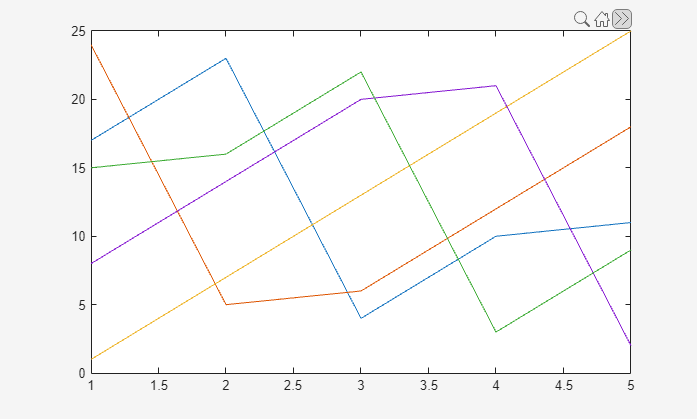```ax1 = subplot(2,1,1); plot(ax1,magic(5)) [tb1,btns1] = axtoolbar(ax1,{'zoomin','zoomout','restoreview'}); ax2 = subplot(2,1,2); plot(ax2,magic(5)) [tb2,btns2] = axtoolbar(ax2,{'pan','datacursor'});```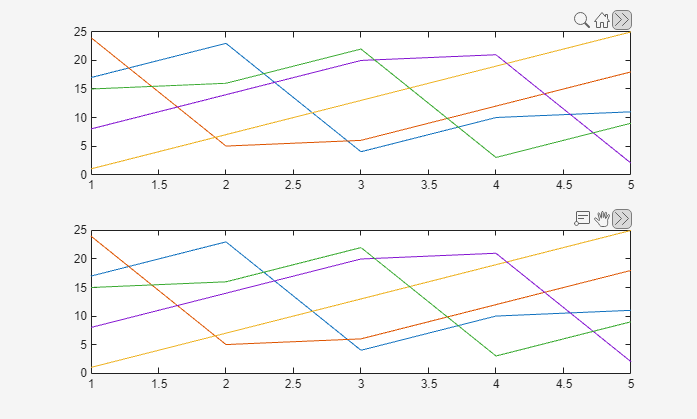```t = tiledlayout(2,1); nexttile plot(magic(5)); nexttile plot(magic(5)); tb = axtoolbar(t,{'zoomin','zoomout','restoreview'});```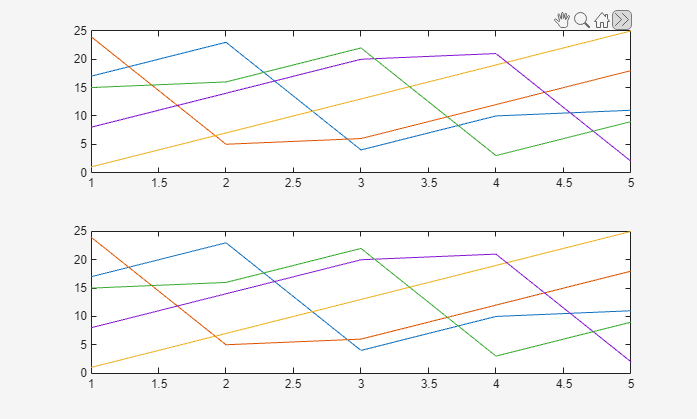## 输入参数

• `Axes` 对象 - 为指定的坐标区组创建自定义坐标区工具栏。

• `TiledChartLayout` 对象 - 创建一个适用于指定分块图布局中所有坐标区的坐标区工具栏。

`'export'`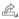`'brush'`切换到数据刷亮模式。
`'datacursor'`切换到数据游标模式。
`'rotate'`切换到旋转模式。
`'pan'`切换到平移模式。
`'zoomin'`切换到放大模式。
`'zoomout'`切换到缩小模式。
`'restoreview'`还原坐标区或分块图布局的原始视图。

•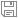：将内容保存为一个精确裁剪的图像或 PDF。

•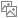：将内容复制为图像。

•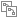：将内容复制为向量图。

### 名称-值参数

• 函数句柄。

• 第一个元素是函数句柄的元胞数组。元胞数组中的后续元素是传递到回调函数的参数。

• 包含有效 MATLAB® 表达式的字符向量（不推荐）。MATLAB 在基础工作区计算此表达式。

`Axes`

`Selection`

`PreviousSelection`

`Source`

`AxesToolbar` 对象

`EventName`

`'SelectionChanged'`

• `'on'` - 显示对象。

• `'off'` - 隐藏对象而不删除它。您仍然可以访问不可见对象的属性。

## 局限性

• 实时编辑器的图窗中不会显示自定义工具栏。要查看自定义工具栏，请点击图窗右上角的按钮以在单独的图窗窗口中打开图窗。